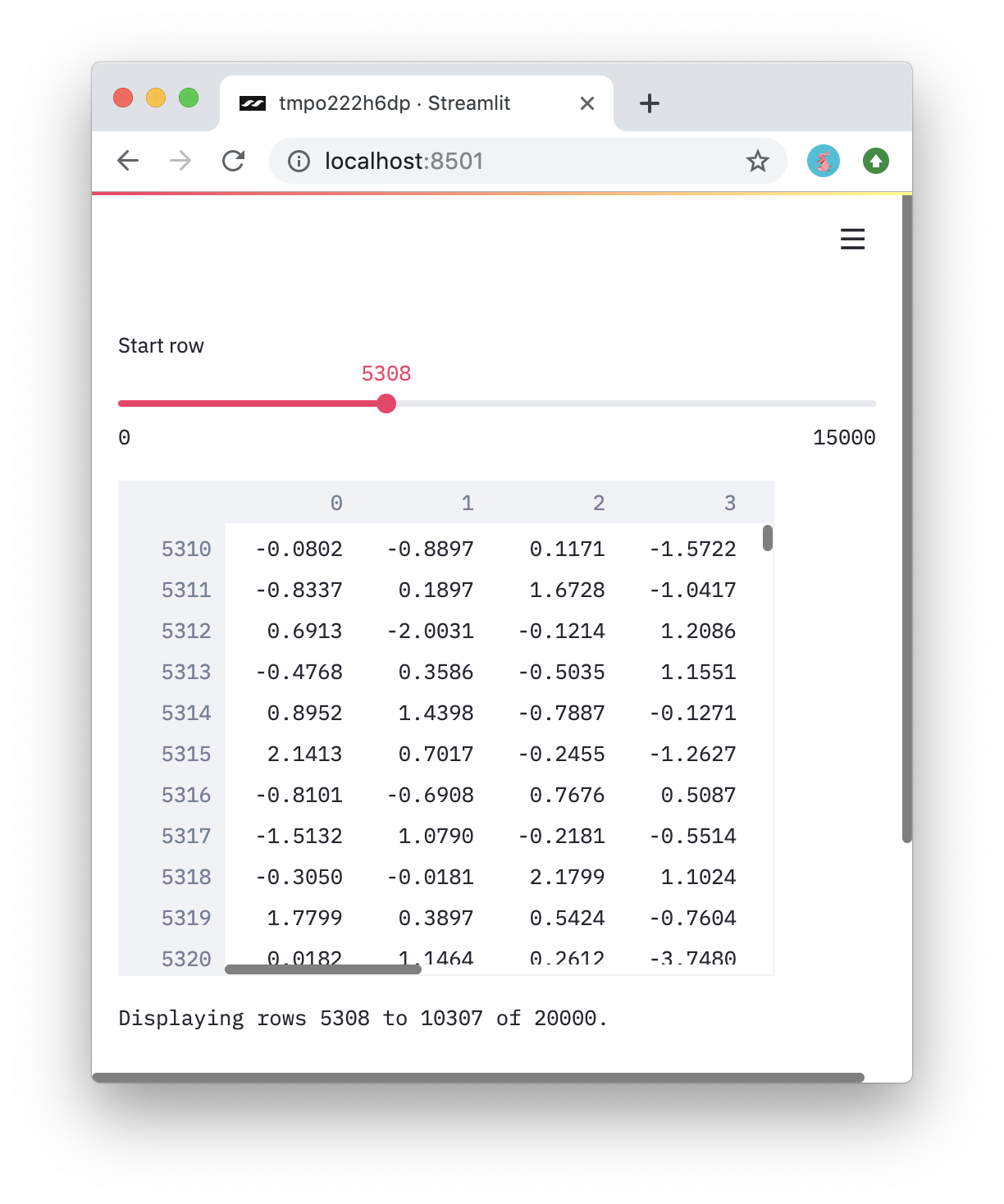Instantly share code, notes, and snippets.

# treuille/display_dataframe_quickly.py

Created October 19, 2019 03:04
Star You must be signed in to star a gist
Workaround: Displaying a dataframe quickly by slicing it.
This file contains bidirectional Unicode text that may be interpreted or compiled differently than what appears below. To review, open the file in an editor that reveals hidden Unicode characters. Learn more about bidirectional Unicode characters
 import streamlit as st import pandas as pd import numpy as np def display_dataframe_quickly(df, max_rows=5000, **st_dataframe_kwargs): """Display a subset of a DataFrame or Numpy Array to speed up app renders. Parameters ---------- df : DataFrame | ndarray The DataFrame or NumpyArray to render. max_rows : int The number of rows to display. st_dataframe_kwargs : Dict[Any, Any] Keyword arguments to the st.dataframe method. """ n_rows = len(df) if n_rows <= max_rows: # As a special case, display small dataframe directly. st.write(df) else: # Slice the DataFrame to display less information. start_row = st.slider('Start row', 0, n_rows - max_rows) end_row = start_row + max_rows df = df[start_row:end_row] # Reindex Numpy arrays to make them more understadable. if type(df) == np.ndarray: df = pd.DataFrame(df) df.index = range(start_row,end_row) # Display everything. st.dataframe(df, **st_dataframe_kwargs) st.text('Displaying rows %i to %i of %i.' % (start_row, end_row - 1, n_rows)) if __name__ == '__main__': # An example of using display_dataframe_quickly. display_dataframe_quickly(st.cache(np.random.randn)(20000, 10), width=400)

### treuille commented Oct 19, 2019

This gist show you a simple method to display a large DataFrame more quickly by showing only a user-specified subset.

To try it, run:

`streamlit run https://gist.githubusercontent.com/treuille/ff9194ed50af277fc56788d7aed7fcba/raw`

You should see this: